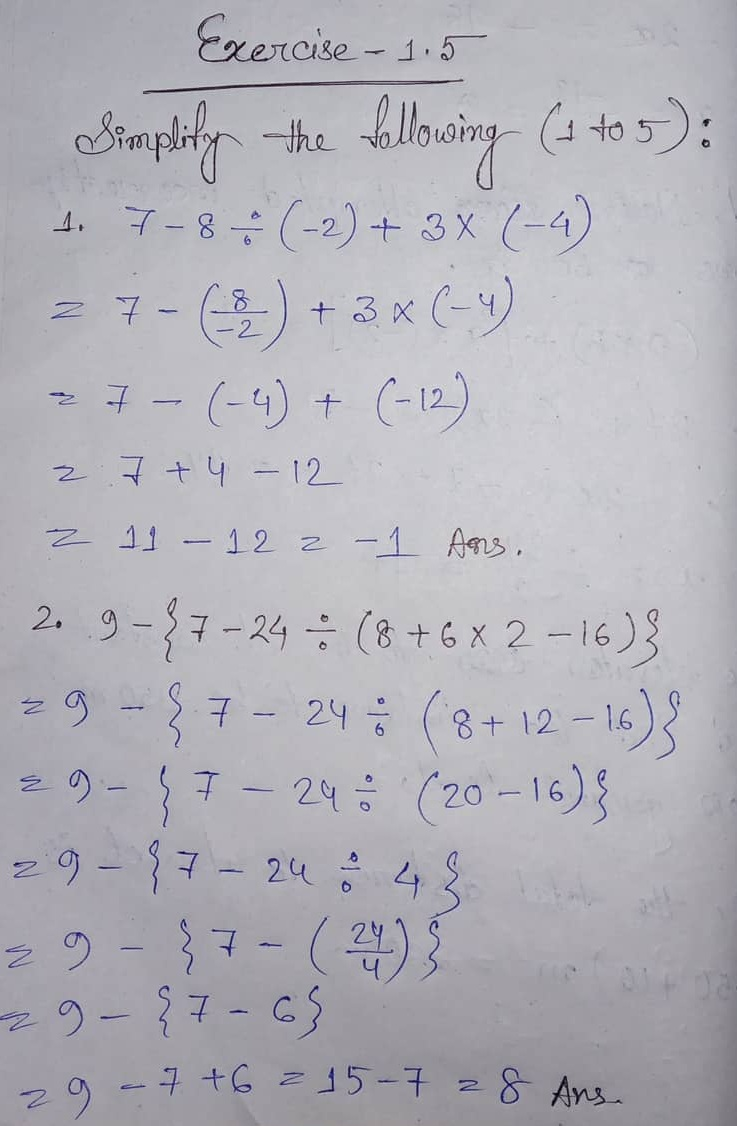# ML Aggarwal CBSE Solutions Class 6 Math First Chapter Integers Exercise 1.5

### ML Aggarwal CBSE Solutions Class 6 Math 1st Chapter Integers Exercise 1.5

Ex – 1.5

(1) 7 – 8 ÷ (-2) + 3 x (-4)

(2) 9 – {7 – 24 ÷ (8 + 6 x 2 – 16)}

(3) 5 x 2 – [3 – {5 – (7 + 2 of 4 – 19)}]

(4) -11 – [-6 – {3 – 5 (8 ÷ 4 – 1)}]

(5) (-3) x (-12) ÷ (-4) + 3 x 6

(6) 14 ÷ (3 of 2 – 3 + 4) – 9 (5 – 3)

### Solution:Updated: October 23, 2019 — 10:56 am

1. sir , where is sixth question.

2. Where is 6 th question